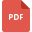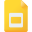# subtraction tutorialsMathematics, Grade 7, United States, SubtractionSubtract Whole NumbersEstimate Sums and DifferencesAdding and Subtracting Fractions Unlike DenominatorsDecimals and Division, Grade 6, United States, Decimals and MultiplicationPolynomial Functions, High School, Addition, SubtractionEstimate Sums and DifferencesAddition, Subtraction, Multiplication, Division of Fractions
Fraction, Grade 5, United States, MathematicsDirected Numbers: Addition and Subtraction WorksheetsEstimating Sum and Difference of Decimal NumbersSolving Equations containing Addition and Subtraction Worksheet